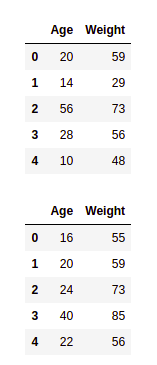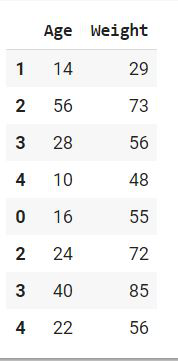Related Articles

# Pandas – Find the Difference between two Dataframes

• Last Updated : 16 Mar, 2021

In this article, we will discuss how to compare two DataFrames in pandas. First, let’s create two DataFrames.

Creating two dataframes

## Python3

 `import` `pandas as pd`` ` ` ` `# first dataframe``df1 ``=` `pd.DataFrame({``    ``'Age'``: [``'20'``, ``'14'``, ``'56'``, ``'28'``, ``'10'``],``    ``'Weight'``: [``59``, ``29``, ``73``, ``56``, ``48``]})``display(df1)`` ` `# second dataframe``df2 ``=` `pd.DataFrame({``    ``'Age'``: [``'16'``, ``'20'``, ``'24'``, ``'40'``, ``'22'``],``    ``'Weight'``: [``55``, ``59``, ``73``, ``85``, ``56``]})``display(df2)`

Output:## Checking If Two Dataframes Are Exactly Same

By using equals() function we can directly check if df1 is equal to df2. This function is used to determine if two dataframe objects in consideration are equal or not. Unlike dataframe.eq() method, the result of the operation is a scalar boolean value indicating if the dataframe objects are equal or not.

Syntax:

DataFrame.equals(df)

Example:

## Python3

 `df1.equals(df2)`

Output:

`False`

We can also check for a particular column also.

Example:

## Python3

 `df2[``'Age'``].equals(df1[``'Age'``])`

Output:

`False`

## Finding the common rows between two DataFrames

We can use either merge() function or concat() function.

• The merge() function serves as the entry point for all standard database join operations between DataFrame objects. Merge function is similar to SQL inner join, we find the common rows between two dataframes.
• The concat() function does all the heavy lifting of performing concatenation operations along with an axis od Pandas objects while performing optional set logic (union or intersection) of the indexes (if any) on the other axes.

Example 1: Using merge function

## Python3

 `df ``=` `df1.merge(df2, how ``=` `'inner'` `,indicator``=``False``)``df`

Output:Example 2: Using concat function

We add the second dataframe(df2) below the first dataframe(df1) by using concat function. Then we groupby the new dataframe using columns and then we see which rows have a count greater than 1. These are the common rows. This is how we can use-

## Python3

 `df ``=` `pd.concat([df1, df2])`` ` `df ``=` `df.reset_index(drop``=``True``)`` ` `df_group ``=` `df.groupby(``list``(df.columns))`` ` `idx ``=` `[x[``0``] ``for` `x ``in` `df_group.groups.values() ``if` `len``(x) > ``1``]``df.reindex(idx)`

Output:## Finding the uncommon rows between two DataFrames

We have seen that how we can get the common rows between two dataframes. Now for uncommon rows, we can use concat function with a parameter drop_duplicate.

Example:

## Python3

 `pd.concat([df1,df2]).drop_duplicates(keep``=``False``)`

Output:Attention geek! Strengthen your foundations with the Python Programming Foundation Course and learn the basics.

To begin with, your interview preparations Enhance your Data Structures concepts with the Python DS Course. And to begin with your Machine Learning Journey, join the Machine Learning – Basic Level Course

My Personal Notes arrow_drop_up